## 7260 Reputation

10 years, 205 days

## MaplePrimes Activity

### These are answers submitted by nm

I think the error  Error, (in PDEtools:-Library:-NormalizeBoundaryConditions)  is a side error, due to using real numbers with exact solver. Maple can't solve this PDE.

Rule of thumb. Do not use real numbers with exact solver. Rationalized all values.  After doing this, the error goes away, and pdsolve returns just () with no solution. Another thing, I do not like using eval to setup BC. Better using D[] directly.

Also, for the Laplacian in cylinderical coordinates, you can use VectorCalculus:-Laplacian to build it, instead of typing it yourself. Less change of error.

Also you had h:=(x,t)->0. But there is no x in this problem. I just set h:=0.

```restart;
a := 35.5*0.0254: a:=convert(a,rational);
b := 36.5*0.0254: b:=convert(b,rational);
L := 0.0254*30*12: L:=convert(L,rational);
alpha := 0.000026972: alpha :=convert(alpha,rational);
Psi_s := 0.0440: Psi_s := convert(Psi_s,rational);
flux_b := 5.1/12: flux_b := convert(flux_b,rational);

h := 0:
the_laplacian_in_cylinderical :=VectorCalculus:-Laplacian( u(r,z,t), 'cylindrical'[r,phi,z] );
pde := diff(u(r, z, t), t) = alpha*the_laplacian_in_cylinderical + h;

bc:= u(r,L,t)=Psi_s,
-alpha*(D(u)(r,L,t))=0,
-alpha*(D(u)(a,z,t))=0,
-alpha*(D(u)(b,z,t))=flux_b;

ic:=  u(r, z, 0) = z*Psi_s/L;

#infolevel[pdsolve]:=5;
sol := pdsolve([pde,bc,ic],u(r, z, t)) ;
```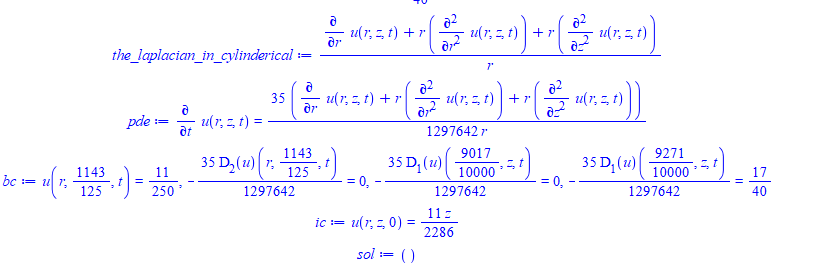You might want to make sure your IC and BC are consistent. You IC says u=z*PSI/L. This means u_z = PSI/L, a constant. This is at t=0, but for all z and all r. But one of your BC says -apha*u_z = 0 (at z=L). Since alpha is not zero, this means u_z = 0 (at z=L). Which is conflicting with the initial condition. I do not know now if this is why the PDE was not solved.

Either way, Maple simply can't solve this analytically. No error generated.

Maple 2020.2 on windows

Your IC and BC are inconsistent.

You have

`ic := u(x, 0) = cos(x), u(0, t) = 0;`

First condition says that for all x, at t=0, u=cos(x).

The second says at x=0, for all t, u=0.

But at x=0, from the first conditions, it says u=1, since cos(0)=1 which is not zero according to second condition.

I could not understand your worksheet. But to solve this pde you could do the following

```restart;
pde := diff(u(x,t),t) = k*diff(u(x,t),x\$2)+h(x,t);
bc  := u(0,t) = 10,u(1,t) = 20;
ic  := u(x,0) = 60*x -50*x^2+10;
sol:=pdsolve([pde,bc,ic],u(x,t))```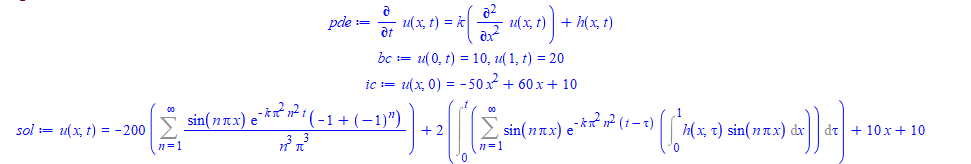For specific h(x,t)

`sol:=simplify(subs(h(x,tau)=0,sol))`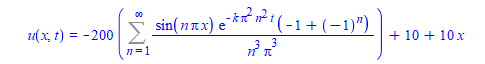you could see example in

https://rosettacode.org/wiki/Associative_array/Creation#Maple

"Maple tables are hashed arrays. A table can be constructed by using the table constructor."

```T := table( [ (2,3) = 4, "foo" = 1, sin(x) = cos(x) ] );
T := table(["foo" = 1, sin(x) = cos(x), (2, 3) = 4])

> T[2,3];
4

> T[sin(x)];
cos(x)

> T["foo"];
1```

if it for display, you could always do

`a*z^``(-1)`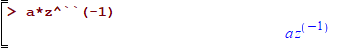I do not do plotting much in Maple, so this might not be the optimal solution. it seems to lose the minor ticks though.  There might be a way to also keep these there. I think the problem is that Maple needs a little bit more space, and so it added ticks by skipping every other value. that is why the odd values 5 and -5 do not show up.

```R:=10:
r:=5:
plot3d( [ ( R+r(u,v)*cos(v))*sin(u),
( R+r(u,v)*cos(v))*cos(u),
r*sin(v)
],
u=0..2*Pi,
v=0..2*Pi,style=patchnogrid,
scaling=constrained,
scaling=constrained,
coordinateview=[-15..15, -15..15,-5..5],tickmarks=[default,default, [seq(i,i=-5..5,2)]],
lightmodel=light3, viewpoint = circleleft);
```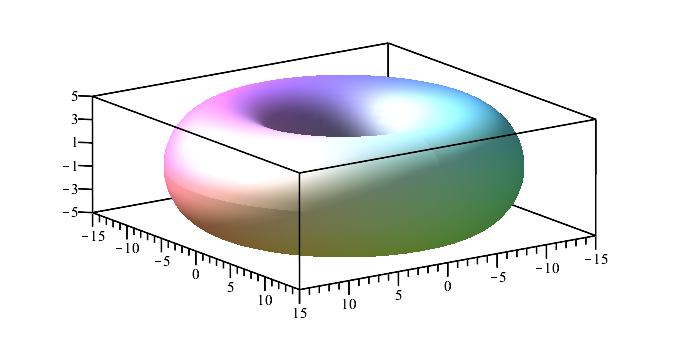Or you could increase the view to coordinateview=[-15..15, -15..15,-6..6] to give it more space?

Maple uses last name evaluation for number of expressions. But this does not apply to things that are rtable based. This includes Matrix, Vector, and Array etc...

last name evaluations is what you are asking for. This means, when you write A:=C; and then assign some value to C, then when you use A again, you expect it to have same value you assigned to C. This is because Maple used last name evaluation to A.

But this does not work with rtables.  That is why when you print the matrix again, you did not see its value changed after you changed m

I do not know if it is possible to change this. But you can always use eval or subs

```restart;
u:=<-2+m,3+m>;
m:=5;
u;
```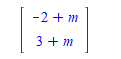`type(u,'last_name_eval')`

false

You can now use eval to force value of m to be used inside rtable

```unassign('m');
u:=eval(u,m=5)```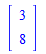I get slighlty different result from that shown.

```f:=t->862.979774+1825.011621/((1+1.54943476*e^(-677.0138344*t))^(131537/203808));
convert(f(t),rational)
```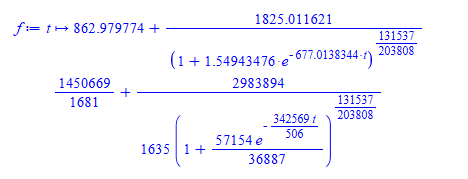Copied the integrand to Mathematica and it gives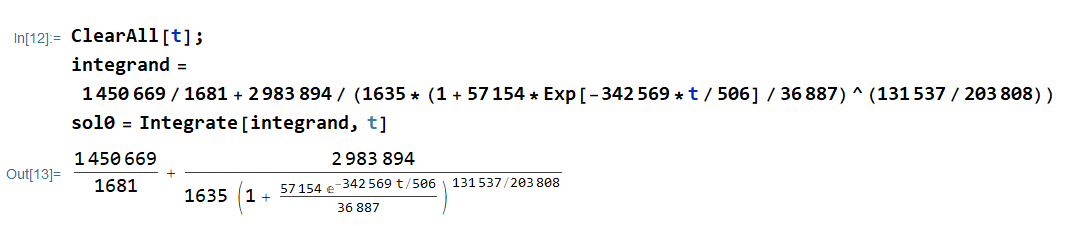Copied the result from Mathematica back to Maple

```expr:=`(1450669*t)/
1681 + (4459704101248*
Hypergeometric2F1[131537/203808, 1, 335345/203808,
(36887*E^((342569*t)/506))/(57154 +
36887*E^((342569*t)/506))])/
(1067737900495*(1 + 57154/(E^((342569*t)/506)*36887))^(131537/
203808))`;
maple_expr:=MmaTranslator:-FromMma(expr);
lprint(maple_expr);

1450669/1681*t+4459704101248/1067737900495*hypergeom([131537/203808, 1],[335345
/203808],36887*exp(342569/506*t)/(57154+36887*exp(342569/506*t)))/(1+57154/
36887/exp(342569/506*t))^(131537/203808)

```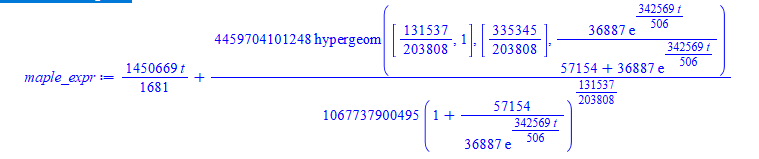Here is plot on top of each others, which shows some difference. I am not sure which is the correct one

```Axel:=2.695678478*hypergeom([1., 1., 1.645396648],[2., 2.],-1.549434760*exp(-677.0138340*t))*exp(-677.0138340*t)+.95671881+1887.991395*t;
plot([maple_expr,Axel],t=-0.01 .. 0.01)```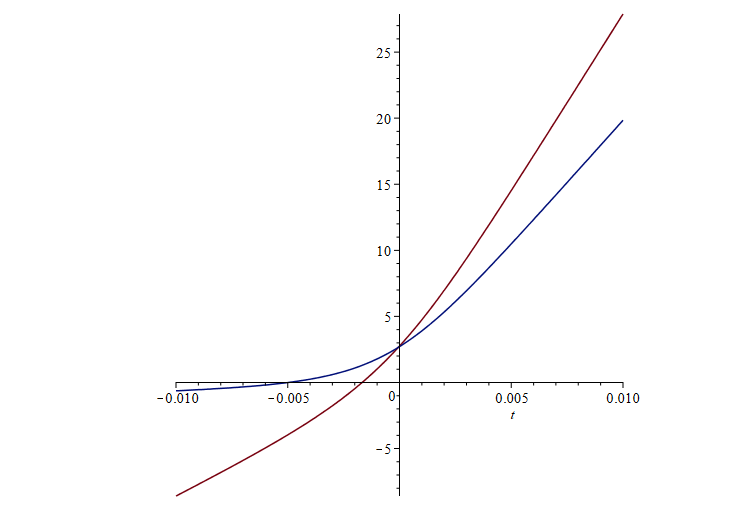How to get time dependence for fixed x?

why do you need to solve this numerically? Maple can give exact analytical answer

```restart;
PDE := diff(u(x,t),t)=1/10*diff(u(x,t),x,x);
IBC := u(x,0)=1, u(0,t)=0, D(u)(1,t)=0;
sol := pdsolve([PDE,IBC]);```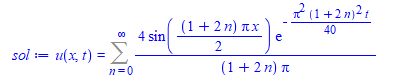To plot for fixed x, with changing t, simply set x to some value in the above, and plot for changing t.

`f:=unapply(subs(infinity=20,rhs(sol)),x,t)`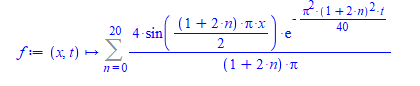`plot(f(1.5,t),t=0..5)`